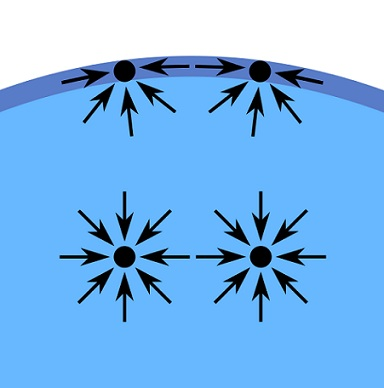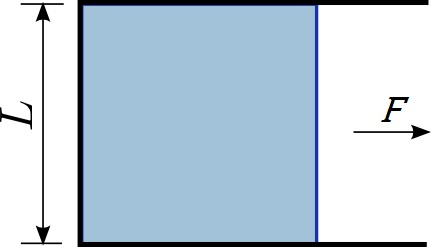# Difference Between Surface Tension and Surface Energy

## Main Difference – Surface Tension vs. Surface Energy

Surface tension and surface energy are measurements of intermolecular forces that make up a material. Due to these intermolecular forces, a liquid surface is always being pulled inward. If one is to stretch the surface, work must be done to overcome the intermolecular forces. The tension on the surface of a liquid and the amount of work needed to stretch that surface can be measured: and these measurements correspond to the surface tension and the surface energy. The main difference between surface tension and surface energy is that surface tension measures the force per unit length of the surface while surface energy measures the amount of work that needs to be done per unit area in order to stretch it.

## What is Surface Tension

Consider a sample of liquid in a container. The liquid is held together by the cohesive intermolecular forces between the molecules that make up the liquid. A molecule inside the container is being pulled in all directions by the other molecules that surround it. However, if you consider molecules at the top surface, the molecules below them are still pulling them down but there are no liquid molecules above them to pull them upward. This means that there is a net downwards force on these molecules. As a result, the entire surface of the liquid is being pulled inward. It is this inward force on the surfaces that causes liquids to form roughly spherical droplets when they are free.Molecules on the surface of a liquid are exposed to cohesive forces from below them. This makes liquid surfaces to be pulled inward.Surface tension causes water to form roughly spherical droplets.

The surface of the liquid acts like a membrane under tension. Surface tension$\gamma$ can be quantified as the force$F$ acting per unit length$l$ of the surface:$\gamma =\frac{F}{l}$

Here, the force$F$ acts parallel to the liquid surface and$l$ is the length over which the force acts. For instance, imagine pulling a film of liquid and stretching it. This is shown in the diagram below:Surface tension on a film of liquid

Here, the force$F$ pulls on the membrane shown in dark blue, stretching the liquid film. Since there are two surfaces in the film (top and bottom surfaces), the total length is$2L$. This means that for this film,$\gamma =\frac{F}{2L}$

## What is Surface Energy

Due to the intermolecular forces between molecules, work needs to be done on a surface in order to stretch it. The surface gains an amount of energy equal to the work that was done on the surface. Surface energy refers to the amount of energy required per unit area to stretch it. For the film of liquid above, suppose the membrane is pulled through a distance$\Delta x$. Then, the work done is$F\Delta x$. The increase in the surface’s area is given by$2L\Delta x$. So, the surface energy$E$ is given by:$E=\frac{F\Delta x}{2L\Delta x}=\frac{F}{2L}=\gamma$

## Difference Between Surface Tension and Surface Energy

### What it Measures

Surface Tension measures the force applied parallel to a surface applied per unit length.

Surface energy measures the energy required per unit area to create a new surface.

Image Courtesy

“Wasser in Tropfen und an der Phasengrenze” by User:Booyabazooka (Own work) [Public Domain], via Wikimedia Commons

“This diagram illustrates the force necessary to increase the surface area…” by Rudolf.hellmuth (Own work) [CC BY-SA 3.0], via Wikimedia Commons (Modified)

“Water droplets on lotus leaf” by aotaro (Own work) [CC BY 2.0], via flickr